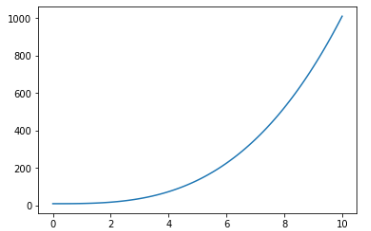# %matplotlib inline

%matplotlib inline是一个魔法函数（Magic Functions）。官方给出的定义是：IPython有一组预先定义好的所谓的魔法函数（Magic Functions），你可以通过命令行的语法形式来访问它们。

%matplotlib inline
import matplotlib as mpl
import matplotlib.pyplot as plt
import numpy as np
x = np.linspace(0,10,100)
y_1 = x**3 + 10
plt.plot(x,y_1)
# 由于 %matplotlib inline 的存在，当输入plt.plot(x,y_1)后，不必再输入 plt.show()，图像将自动显示出来12345678©️2019 CSDN 皮肤主题: 大白 设计师: CSDN官方博客